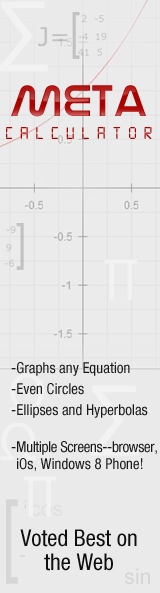﻿ Triangle Worksheets- Free pdf's with answer keys on triangles

Triangle Worksheets

Geometry

These worksheets focus on the topics typically covered in Geometry

Ultimate Math Solver (Free)

Free Algebra Solver ... type anything in there!

Math Worksheets Go! Free Printable Math Worksheets!

Free Printable Math Worksheets with answer keys

Enjoy these free printables. Each one has model problems worked out step by step, practice problems, as well as challenge questions at the sheets end. Plus each one comes with an answer key.

 Search for a TopicWorksheets by Topic

 MathWorkSheetsgo.com recommends :-a powerful graphing calculator that can graph cool things like sin(y) = cos(x) !!